Categories

## What makes an equation tricky to solve?

Here are four equations, each using similar numbers. Which one would be the most difficult for a student to solve?Conventional wisdom is that this question is too complex to really grapple with; it depends on the kids, the class, what they’re studying, etc.

I think the conventional wisdom has a point, but I know that I’ve learned a lot about the differences between these four problems, and there ought to be a way to teach what I’ve learned to others. Of course, only other people can be the judge of whether I know something worth teaching! Here’s a rough attempt to get at some of the pedagogical structure that underlies these problems.

## Equations that can trigger arithmetic

Part of the problem with trying to make generalizations about how kids will tend to struggle or succeed in solving equations is that there are MANY ways to successfully solve an equation. I can’t say, “when kids see bla bla bla they do bla bla bla,” because just as many kids will as won’t do that, and it largely depends on the approaches to instruction that the teacher takes with the class.

So I want to play with the idea that it can be helpful to think of an equation as having triggers that can activate various strategies that a kid might potentially use for an equation.

Here’s at attempt to explain what I mean in the context of the four equations above. There are many different ways that a child might think about solving x – 12 = 9, especially after receiving instruction on how to solve these types of equations from a teacher. Here are just a few:

• An “undoing” metaphor which involves adding 12 to the right side
• A “balanced moves” metaphor which would involve adding 12 to both sides
• Just to get weird: a kid could multiply both sides by -1, add x to both sides, then perform 12 – (-9).

I’m sure there are many more potential strategies out there. What I think is special about x – 12 = 9, though, is that it is especially well-suited for one way of thinking, one strategy, in particular:

• Just treat it like an arithmetic problem — what minus 12 is 9?

The idea I’m playing with is that the equation contains a trigger for using an arithmetic strategy, i.e. a strategy that depends on no new algebraic metaphors, procedures or ideas, just whatever knowledge of the operations and arithmetic a student comes into this work with.

I want to be clear about two things. First, I’m not claiming that all students are going to approach this equation using arithmetic. Instead, I’m saying that these equations are especially well-suited for arithmetic, and a student who uses arithmetic sometimes to solve equations would very often use it in x – 12 = 9.

Second, I think that arithmetic is generally something that students have had a lot of experience in, compared to algebra. By the time an 8th Grader gets to an equation like x – 12 = 9, thinking about this equation in terms of arithmetic is likely to be productive and yield correct and helpful thoughts about the equation.

So, here’s a big generalization that I want to throw out there: equations that can trigger arithmetic are going to tend to be easier for kids.

## Not all arithmetic is equal

One of my favorite things in math education thought is CGI. CGI stands for something, but I don’t really care about “Cognitively Guided Instruction.” What I love is the way it so convincingly analyzes the different strategies that young children will tend to use for various arithmetic problems. Here is an excerpt from a wiki article I wrote on CGI:Their earliest work identified a taxonomy of addition and subtraction word problem types. Different problem within this taxonomy are differently difficult for children even when the “arithmetic” remains constant. For example, “Tom had 11 apples, and then lost 3 of them, how many does he have left?” might be easier for a student to represent than “Tom has 11 apples and 3 of them are spoiled, how many are not spoiled?” as the former problem has an action that a student can act out using counters, paper or some other representation.

Different arithmetic word problems trigger different strategies, even when they use the same numbers. And they have a systematic way of thinking about it!

(I hope it’s clear that they’re my paradigm for the sort of systematic approach I’m trying for here…)

Here is my understanding of the way arithmetic works. Kids form meaning of the operations in terms of contexts and word problems that are naturally associated with actions. So, subtraction gets associated with taking stuff away , and that meaning is there even when the contexts aren’t. Attempts to give kids alternate ways of seeing the operations are hard precisely because the operations are built so snugly on these paradigmatic actions. This determines which strategies get triggered by various subtraction problems, even without contexts, and even when they contain the exact same numbers.
And here’s a particular example, connected to the equations above: x – 12 = 9 and 21 – x = 9 are not the same type of problem. The first has the start as an unknown (if you think about it as an arithmetic problem, aka with the paradigmatic meaning of something takeaway 12 is 9); the second has the change as the unknown (aka there was 21 and then some got taken away and now there’s 9).
Neither equation is particularly easy to think through using arithmetic, but there’s a strategy available to young students for 21 – x = 9 that isn’t available for x – 12 = 9. To solve 21 – x = 9, you can go down from 21 until you get to 9, i.e. 21 minus 10 is 11, minus 11 is 10, minus 12 is 9, got it! 12!
You can’t really do that for x – 12 = 9 because you don’t know where to start. You’re left with trial and error. Of course, trial and error was an available strategy for 12 – x = 9 too, so there are fewer approaches available for x – 12 = 9.
In other words, if you think about it in terms of arithmetic, x – 12 = 9 is probably harder for students than 12 – x = 9.

## Equations that don’t trigger arithmetic

But of course most equations can’t be solved easily by thinking of their paradigmatic meaning from arithmetic. Often you can de-trigger arithmetic in an equation by messing with the numbers, and intimidating any kid’s sense that arithmetic might be productive:

x – 12.05 = 9/2

You could think about this as arithmetic, but the arithmetic would not be a friendly way to go about this since the numbers are not so amenable to the strategies and experiences of arithmetic. That’s a precise sense in which this type of equation is more difficult — arithmetic would be unavailable to students who might solve it, and they’d have to rely on newer, more algebraic approaches.

You can also de-trigger arithmetic by tossing in more variables, but here some subtlety is needed:

21 – 10x = 9

It seems likely that students would not tend to use arithmetic naturally on this because it’s so much more complex looking than a typical arithmetic problem. At the same time, kids can learn to use their arithmetic on this type of problem, and for a lot of kids this can be productive as they’re getting the hang of algebra.

If a kid is comfortable with arithmetic, they could think of this as 21 – BLA = 9. BLA must be 12, so 10x must be 12, so x must be a tenth of 12, or 12/10.

Some people call this move “covering up” or “chunking” the equation, but the point is that it extends the power of arithmetic to apply to a greater class of equations.

Precisely because 10x – 12 = 9 is tougher on the arithmetic, it’s less likely for “covering up” to be helpful on this problem. So this a precise sense in which 10x – 12 = 9 can be tougher than 12 – 10x = 9 — there’s a strategy for one that the other doesn’t have.

That said, there are other interpretive issues that could arise for 12 – 10x = 9 if you use a more algebraic technique that wouldn’t arise for 10x – 12 = 9. In particular, the metaphor of undoing or unwinding (i.e. inverse operations) fits more naturally with 10x – 12 = 9 than 12 – 10x = 9. So that’s another precise sense in which one of these equations is trickier than the other.

In practice, I’d predict that 12 – 10x = 9 is easier for students very early in instruction who would be more likely to be triggered to use arithmetic, whereas later in instruction when kids have learned algebraic techniques 10x – 12 = 9 would be easier, because it fits better with the unwinding/undoing way of seeing an equation.

So, in short, not only are equations that have arithmetic triggers maybe easier, but equations that can be covered-up to allow arithmetic to hook-up with a student’s thinking will also be easier.

(Another subtle distinction: 12 – 2x = 9 allows for easier arithmetic than 12 – 10x = 9 because you can use your arithmetic to solve 2x = 12 but it’s tougher to use arithmetic for 10x = 12.)

## Texts and teachers don’t make these types of distinctions

There are a few other dimensions that I think equations differ in important ways. Here are a couple that might make for good other case studies, if this becomes a series of posts:

• Equations that trigger problems with managing zero (like 2x – 5 = 7 – 3x)
• Operations that fit naturally with a “balancing” metaphor (adding/dividing, maybe multiplying) and operations that don’t fit as naturally (subtracting)
• Distributive property equations that are amenable to “covering up” vs equations that aren’t

This is rarely (never?) the way equations are presented to kids in texts or by teachers, though. I think the dominant approach is to classify problems by surface-level complexity and to vary everything else, in the hopes that kids will get exposure and practice with all these different types of problems. My point is that there is no organized, principled, systematic way that we have of thinking about the various different problem types of equations, so we just typically put them all in a blender and then present them to students all at once. And then we spot-check the difficulties for months and months.

And my theory is that if we have a theory of the problem types and micro-skills that give equations their underlying pedagogical structure, we might be able to design better resources and teaching for kids in this skill.

And my other theory is that if we’re attuned to this sort of thing, even if we don’t have a system, meaning if we’re looking out for these sorts of problem types and micro-skills, we can generate better feedback and responses to the stuff we see kids have trouble with in class and on assessments.

Categories

## Dear Diary: What types of equations are hard to solve?

I’ve been begging people lately to send me their solving linear equations mistakes. As long as people indulge me, this will be a series of posts devoted to thinking aloud about what sorts of linear equations are hard to solve, and what it is that makes them hard, and what exactly it means to know how to handle every equation that’s out there. The goal I have in mind is a way to be able to teach a (relatively) new teacher what sorts of equations they can expect their students to struggle with and (ideally) how to systematically teach this stuff so that kids see all the cases. (Besides for the obvious, e.g. an equation with lots of stuff in its that requires a ton of steps).

I don’t have settled thoughts about this, but I do need a place to work this stuff out. These posts are unfinished and sketchy. It’s basically my math mistakes diary. You should only read this if that sounds like fun to read…

…and here we go!

***

I think there are a lot of things that make this hard. I don’t know about Michelle’s students, but fraction multiplication is touch-and-go with my students, so there’s that factor.

One of the most important little “micro-skills” relating to equations that I teach my students is about scaling equations up so as to eliminate fractions. I think that would be useful here.

There’s an interesting choice about whether to do that scaling before or after distributing. That’s another interesting little choice that a student would have to make. I could imagine designing a little activity centered on a bit of strategy: when does it make sense to scale up? I think the message to kids could be that if you scale up before distributing, you might get to avoid some fraction arithmetic.

Maybe the activity could look like this. You start with that equation and then you present two options.

“Amanda took that equation and did this: 14m – 7 – 3m/5 = 24/5 – 18m/3”

“Billy’s first move looked like this: 35(2m – 1) – 3m = 6(4 – 3m)” [include annotations to make it clear what Billy did!]

And then you offer some prompts to draw attention to the choices you get to make?

Micro-skills here: knowing whether to distribute first, or whether to wait; scaling up an equation to avoid fractions

***

Awesome!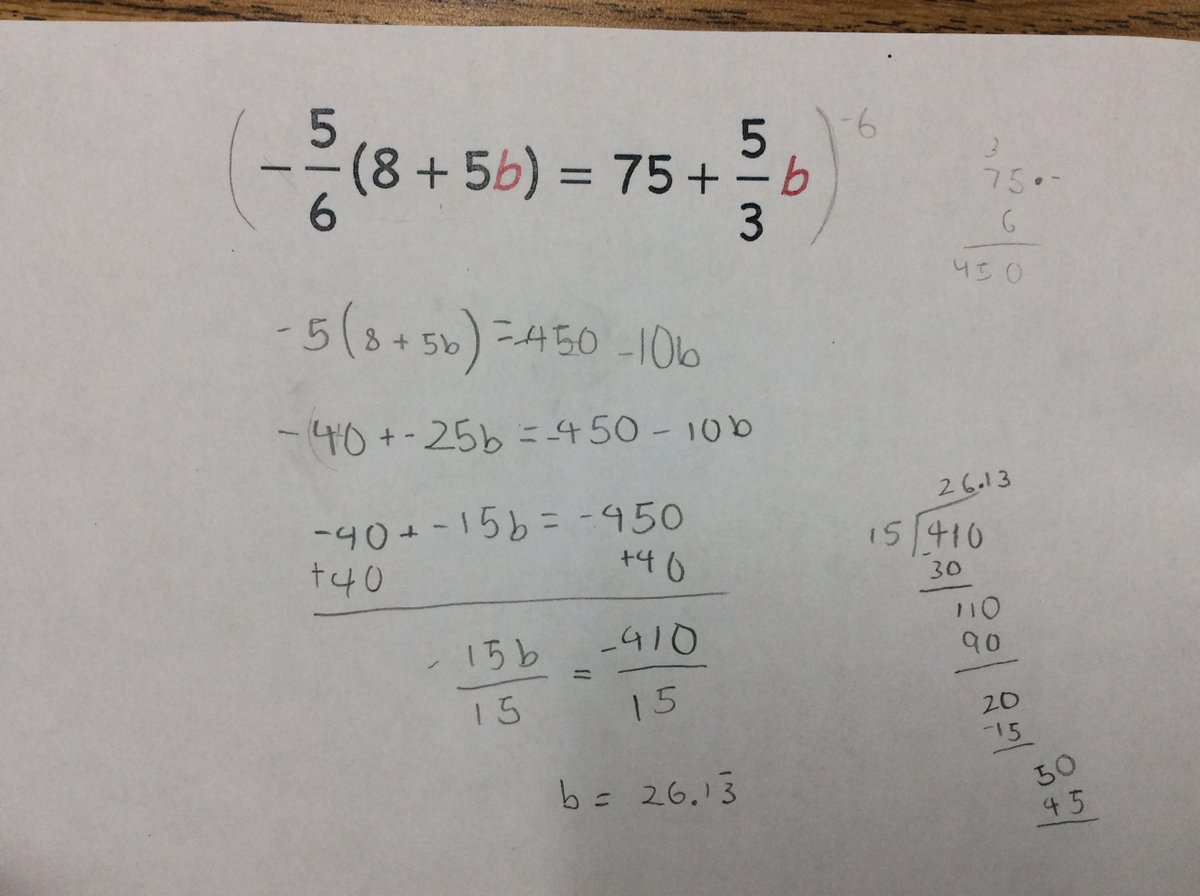Just be explicit about the mistake…we scale both sides by -6, but the left side ends up only getting scaled by 6. The fractions seem sort of incidental to this one. Maybe this is related to the thing where multiplying by a negative feels like it should make the stuff negative, rather than giving everything its opposite sign?

To what extent is this an equations mistake vs a negatives mistake?Lots going on here. The idea that we’re scaling both sides vs. just the fractions is tricky it seems. Clearly fractions make an equation tricky to solve as it presents a number of conceptual issues. To what extent is it helpful for a teacher to understand anything about how kids handle equations with fractions besides “with difficulty”? It seems like all the interesting choices to make when handling equations with fractions are about finding ways to transform that equation so that we can avoid those fractions.There’s an interesting choice to make about whether you try to multiply both sides of an equation by 2 or by -2. What makes that interesting is again that multiplying by a negative introduces conceptual issues that aren’t introduced by x2. I feel like I’m getting close to saying that, as a matter of coaching, it makes sense to encourage kids to scale equations by positive numbers whenever possible. (Which, I think, is always.)

Micro-skills involved: What do you choose to scale each side of the equation by? When do you choose to scale each side of the equation? (Do you distribute first, or scale first?)

***

This was one of mine that I wrote about elsewhere:

Yesterday I gave students a no-grades quiz in algebra. A student who, I had been told at the start of the year, frequently struggles in math, has been having a lot of success lately. She knew exactly how to handle both of the systems of equations that were on this short quiz, but she got stumped at one of the resulting equations:$-1.7x = 4.3x + 3.6$

I didn’t know what to say when she got stuck, exactly, but I was fairly confident that this was an example of a micro-skill that she was missing.

She and I agreed that she’d like me to write a little example on the side of her page, so I wrote this:$-2x = 5x + 7$

[I drew some arrows going down from each side labeled “+2x.”]$0 = 7x + 7$

My student read the example and then exclaimed (in a way I can only describe as “joyous”), Oh wait, you can make 0 there?!

Micro-skills: Knowing that you can make 0.

There seem to be a bunch of cases where you introduce and manage zeroes in solving equations. Another one of mine that I have rattling around in the archives:Check out the bottom right. This pair of students got frozen at y = 2y + 5 because they subtracted y from each side and then weren’t sure if they were allowed to end up with 0. (Two years ago I talked to these kids about this, so I’m not guessing from their work.) This seems identical to the situation that this year’s student.

The moral of the story to me is that making zeroes is something that students need “permission” to do. Not like permission from me, the mathematical authority. I just mean that it seems to be something that beginners need help realizing is a kosher move.

As long as this picture is here, another little decision that kids need to make (see top-right) is whether to add or subtract something when trying to use a balancing move.

Microskill: “making a zero” to decide whether to add/subtract something from each side of equation

Categories

## Constructing Trinomials – Where to go Next?

Hi everyone,

My name is Bryan Penfound. Awhile back I was asked if I would be interested in helping out at MathMistakes and I said yes not knowing how challenging this term would be for me. Now that I have settled in a little bit, I thought I was a bit overdue for a post, so here goes!

Recently while volunteering at a local high school in a grade 9 classroom, I had to opportunity to observe students’ answers to the following question: “Create a trinomial in the variable t that has degree 3 and a constant term of -4.”

Here are five of my favourite responses:I would love to get some discussion going. Choose one of the polynomials above and try to deconstruct what the student knows and what the student still has misconceptions about. What follow-up questions might you ask to learn more information about how the student is thinking? What follow-up questions might you ask to help with any current misconceptions?

Categories

## Which Activity Would You Choose?

In an earlier post, I shared Michael Fenton’s scenario and categorized the responses he got on twitter.

There were at least seven distinct responses that teachers offered to Fenton’s prompt. Wow! This makes me think two things:

1. Fenton’s scenario was so thought-provoking that it yielded an amazing variety of responses.
2. How come there was so much disagreement about how to act in this scenario?

Part of the disagreement, I think, comes from what went unspoken in Fenton’s mistake. We didn’t know if this mistake was shouted on in a discussion or found on a piece of paper. We don’t know if this is one of those times when we can afford to have a one-on-one conversation with a kid in response to her mistake, or if our response will be scrawled on her paper and returned. Was this a common error, or an isolated mistake? Could our response be an activity for the class instead of a chat?

While one-on-one conversations are crucial in teaching, they are hard to talk about. By their nature, they’re improvisational and somewhat unstructured. I’d also argue that opportunities for one-on-one conversations can be rare, and they get rarer as the number of students in your class grows larger.

Revising the Scenario

So let’s add some details to Fenton’s scenario. This was a mistake in an Algebra 1 class. Smart kids, thoughtful teacher, but when she collects papers after an ungraded check-in she finds that about half her class made Fenton’s mistake. Oh no! She decides that she’s going to launch class the next day with a brief activity to help advance her kids’ thinking.

Her first idea is to try a string of equations. She has three different drafts. Which one would you choose, and why?

Equation String 1Equation String 2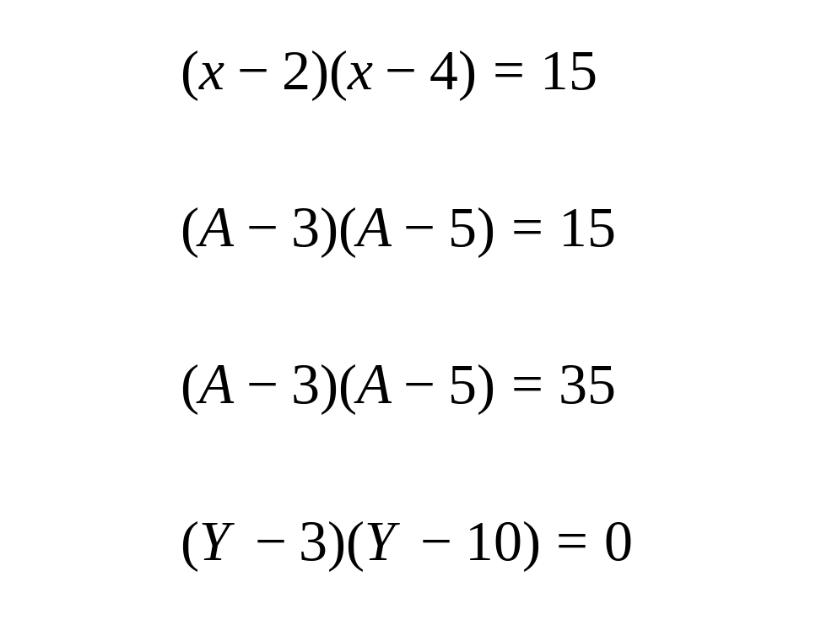Equation String 3Other Activities

Then, she has some other ideas. Maybe equation strings aren’t the right move? She comes up with three other activities: Working With Examples, Which One Doesn’t Belong and Connecting Representations.

Working With ExamplesConnecting RepresentationsWhich One Doesn’t Belong?Commentary

The meta-question here is about the conversation. Can we have a conversation with so many options? I don’t know. I worry that maybe I should have just limited discussion to the equation strings.

What would do?

My first reaction is that I like the equation strings, because it most directly gets at the issue of overextending the zero-product property to other equations.

But what I really want to do is lay out a sequence of 3-4 activities that I could do in sequence to develop this idea for a class.

And do I know enough to answer that question? Wouldn’t that depend on the math that we’d already studied and the math that’s coming up next?

Do we learn anything from thinking about these questions?

Categories

## How Teachers Would Respond to A Quadratics Mistake

Lots of responses to this great tweet. I wanted to understand the themes in what people were replying, so I went through everything and tried to summarize it here.

Response #1: Check Your Work, Start a Conversation

Response #2: Just Check Your Work (No Conversation Mentioned in Tweet)

Response #3: Explain the Zero Product Property

Response #4: Thinking About How to Teach the ZPP Unit

Response #5: Switch to a Graphical Context

Response #7: Run a New Activity with the Whole Class

I’m sure I didn’t capture everyone’s response, and I don’t know what any of this means. But there you go.

Categories

## A Class Set of Identities Work

Predict: What responses to this prompt would you expect from my Algebra 1 students? (Prior to this problem my kids had mostly worked with integer arithmetic, solving linear equations in one-variable and graphing scenarios and equations.)Study: What do you notice in this (small) class set of responses? Note anything that surprises you.

Kid 1:Kid 2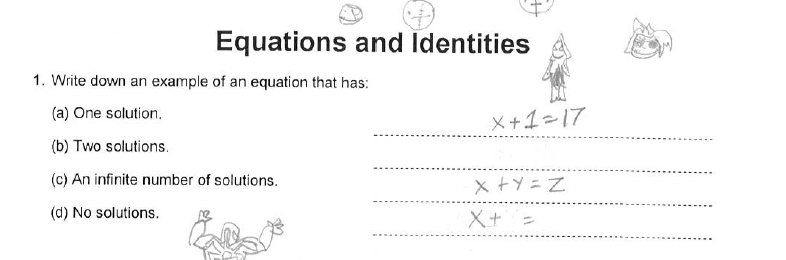Kid 3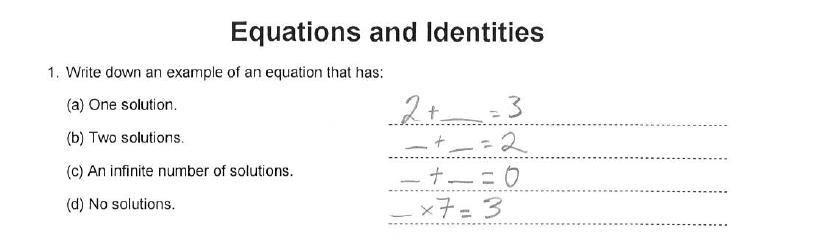Kid 4Kid 5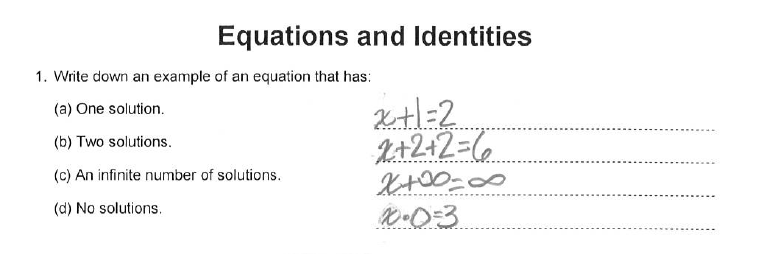Kid 6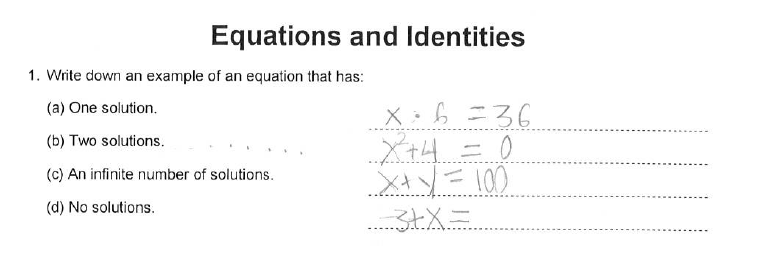Kid 7Kid 8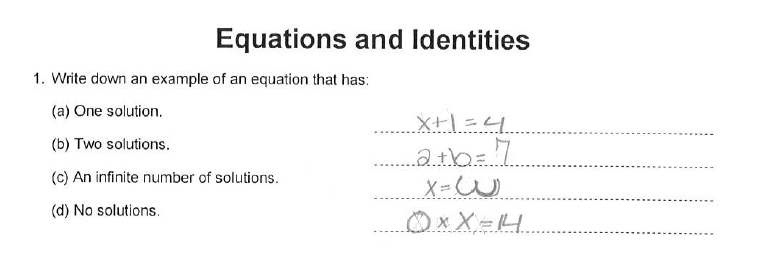Kid 9Wrap Up

How did your predictions hold up? What surprised you the most? What’s something you wish you knew more about?

Categories

## Recursive and Relational Thinking and the Feedback each DeservesTake a moment  before reading on. How many squares would be in the 7th step of this pattern? In the 43rd? In the nth?

Take another moment: what mistakes would you expect to see?

From looking closely at student work with other visual pattern problems, you’d expect kids to think about the change of this pattern in two different ways.

1. Thinking about the pattern change recursively – Students would think about the pattern as adding four squares on to the previous image at the corners.
2. Thinking about the pattern change relationally – i.e. by relating the step number to some part of each picture (e.g. number of squares in diagonals,  number of sets of four squares on the corners, etc.)

The relational goggles are more powerful and useful. Unit Chefs helps us calculate any step of the pattern efficiently. It can be generalized to linear functions. Further, most students have an easy time seeing this pattern’s recursive growth. The real learning that can happen with this pattern, for most students, happens in the move from a recursive to a relational perspective.

With that in mind, I want to share some mistakes that my students made on this pattern. I’ve organized the mistakes into two categories, and I’m curious if you’ll see them the way I do.

Category 1: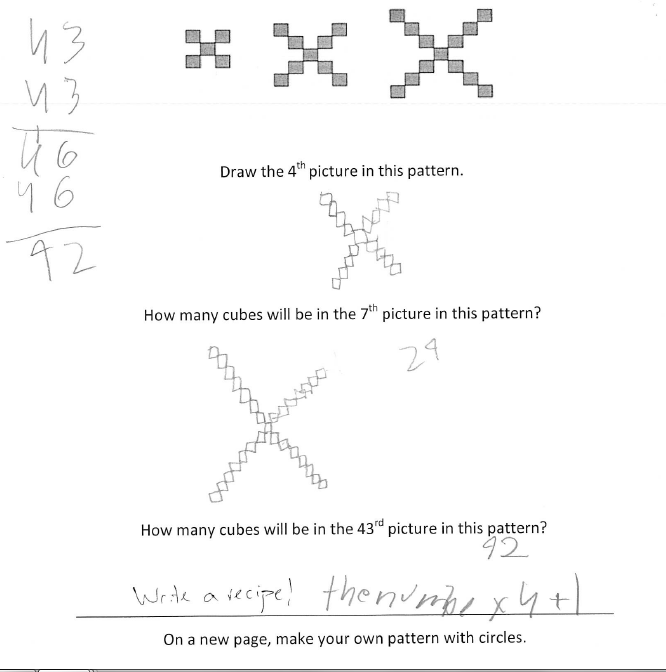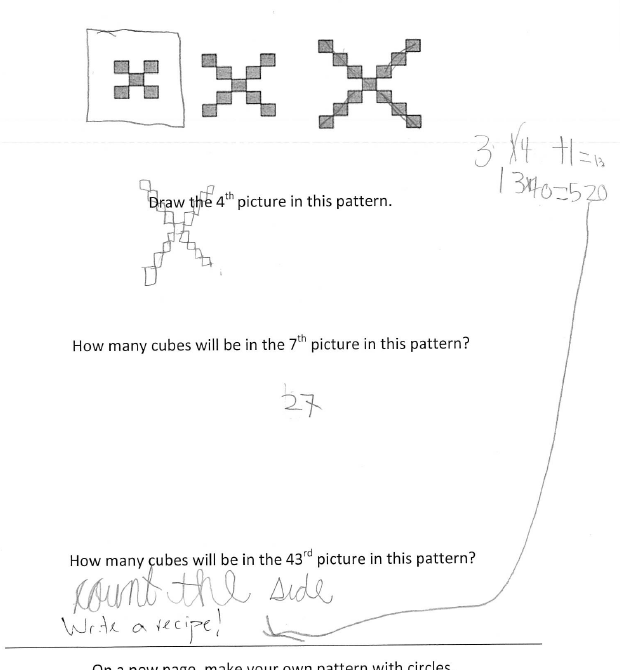Category 2: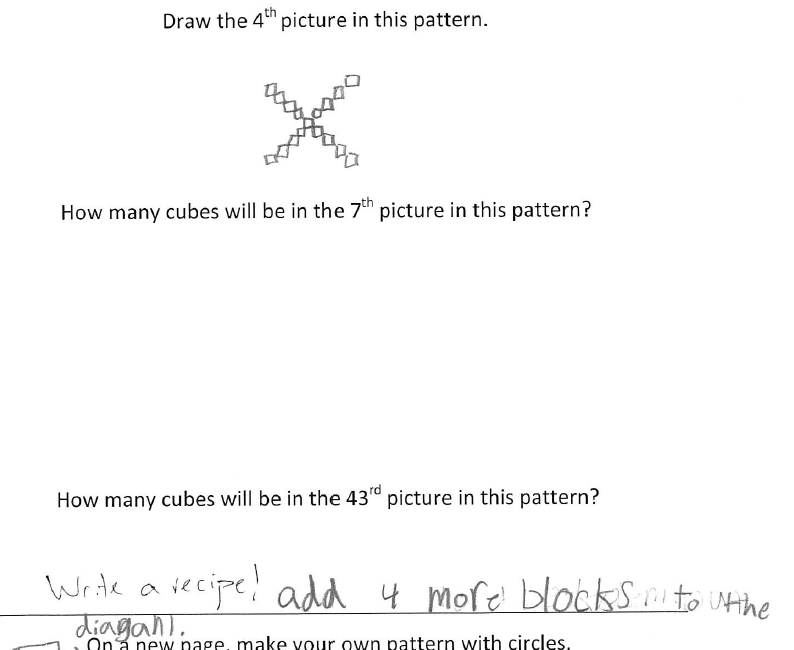The way I see it, all the mistakes that I placed in Category 1 show strong evidence of seeing the pattern’s change relationally. Both of the students in Category 2 show a recursive perspective. In fact, the students in Category 2 don’t even make any mistakes!

What feedback do you think the students in Category 1 should get? What about the students in Category 2?

If all you care about is whether a student’s answer is right or wrong, then all the students in Category 1 will get some sort of nudge towards the right answer, while the students in Category 2 will be praised for their correct answers and maybe encouraged to keep on going.

But the students who are able to relate the step number to part of each picture are actually in pretty great shape. Yeah, they made some mistakes, but most of those mistakes are “off by 1” or “sloppy errors,” the sorts of mistakes that are almost always the result of paying attention to something besides the calculation or step number. (In this case, attention is being sucked up by the need to focus on the structure of the pattern at each step, a way of thinking that is brain-consuming when it’s new.)

On the other hand, the second group of students are getting right answers using a limited perspective. Ultimately, we’d like to help them see a relational perspective. Even though they have the right answers, they’re struggling here.

It’s not news that kids who get the wrong answer might be thinking in more sophisticated ways than students who got some question correct. What is news, I think, is that we ought to be as explicit as possible to ourselves about how those students are thinking with more sophistication. That’s the sort of thinking that can help us be strategic about the sort of feedback that we can give.

What feedback should Category 1 get? I’m inclined to use a very light touch with these students. They’re working within a powerful framework — they’ll likely be able to tease out where they went wrong. Even though they are using a strong perspective to analyze the problem, I still think it’s worthwhile to ask them to correct the calculations. First, because even though getting a correct answer isn’t all that matters, it also matters to students and to me. I want to show that I value correctness. Second, because seeing what doesn’t need to change in their answer is ultimately good for learning. I see this as a chance to adopt that relational view on the pattern again (“Oh wait how did I do this…Oh yeah!”).

Here are some comments I’d give Category 1 kids:

• I love the way you brought the step number into your calculation.
• Can you revisit this? Something’s wrong, but I’m not sure what.
• Your rule here is excellent. Can you check these answers again?

Some teachers will be tempted to encourage Category 2 students to continue their work, even if it’s within a recursive perspective. They might agree that the goal is ultimately for these students to adopt a relational perspective, but they’re willing to bet that kids will come to a “realization” while working recursively all on their own. Or, teachers want to affirm these students’ good thinking, so they are reluctant to offer them another way of thinking. They’re willing to defer the relational view to some other time, and maybe the kid will just pick up the relational view during a class discussion or by talking with a classmate.

Those are all legitimate moves, depending on the kid and the classroom and the course. But what if it’s important — for the kid, classroom, course — to help these students move from a recursive to a relational perspective? What feedback could they get then?

For these students, we want to offer them a new way of thinking. Here’s what I might say:

• Lovely work so far. Can you see where the step number appears in each diagram, and use that to find the 43rd step?
• I see the 4th diagram as made up of 3s. Can you see it as made up of 4s? Try to use that to find the 43rd step.
• Nice job noticing the growth pattern. Can you find a solution to the 43rd step that doesn’t involve adding 2 forty-three times?
• Can you show that there’s a counter-example to the “multiply the step number by 4” rule?

Any other ideas, people?

I’ve squawked a bunch about feedback. I’ve likewise done my share of squawking about student mistakes. I’m realizing now just how much that squawking has been missing out on by failing to get specific about student thinking. This isn’t the familiar complaint (familiar to me, at least) that by focusing on mistakes we only see students for their errors. Or maybe this is that “deficit model” complaint, but I had always interpreted as saying something about what we value in our students, and now I’m seeing how only thinking about mistakes really gives you nothing to latch the errors on to. It’s really limiting.

The flipside of this realization is that to really get at mistakes, feedback, hints or next instructional steps, we need to map out the terrain of student thinking. And there’s no way to do that without looking at sets of student work, rather than some single kid’s  thinking. And there’s no way to do that without getting messy with the details of particular mathematical topics.

This is as true in my teaching as it is for my work here or anywhere else. My best feedback comes when it’s purposefully guided by some sort of explicit story about how student thinking develops for this type of problem. This is probably something I first really learned how to do with multiplication in 4th Grade, and it’s heavily influenced by the way I read the work of the Cognitively Guided Instruction team.

This post is a long, long way of saying that while I’d still love it if you send in individual mistakes that tickle your fancy in any way, I would LOVE it if you could send me a class set of really anything that your students have done, and especially if it’s from a geometry unit or a geometry class. I would be eternally grateful for your class scans: michael@mathmistakes.org. (I’m really good at quickly anonymizing student work.)

Next post: more on why class sets are the best.

Previously: http://mathmistakes.org/visual-patterns/

Categories

## What feedback would you write for this quiz?

In a lot of ways, it’s much easier for me to come up with helpful feedback to give on rich, juicy problems (see here) than it is for your typical quiz or test. I find it much harder to think about how to give feedback that helps a kid’s learning when (a) the quiz is full of non-open questions and (b) the kid’s solutions don’t show a lot of thinking. But a lot of classroom assessments end up like that, and it’s important to figure out how to deal with those tough situations effectively.

So: What would you write as feedback on this quiz?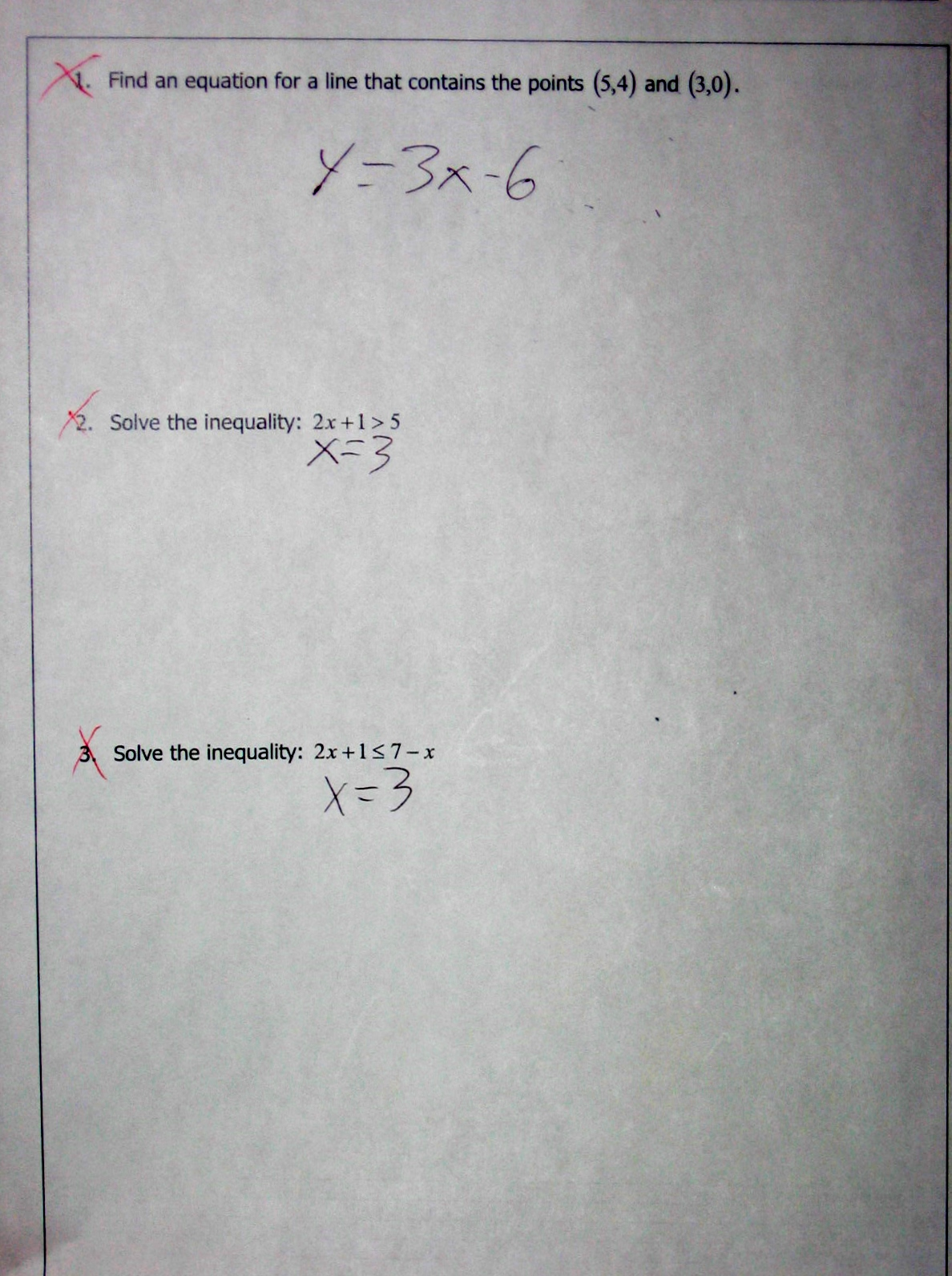Some constraints/notes, that you should feel free to reject or challenge:

1. Assume that we’re dealing with written feedback here. Not a conversation.
2. Assume that we don’t have to write a grade on this piece of work. (If we wrote a grade on here, some research indicates that would ruin any feedback we gave.)
3. You might decide to give feedback on every question of this quiz, you might not.

• Would you choose to mark the questions as right/wrong?
• Would you ask questions or give suggestions?
• Would you write one, several, or many comments?
• Would you reject the constraints in some way?
• Would you ask the kid to explain himself?

Categories

## ln(5) – ln(4) = ln

ln(5) – ln(4) = ln

Thanks, Jack!

Categories

## Squaring doesn’t make equivalent fractionsSquaring doesn’t make equivalent fractions.

Thanks again to Gregory Taylor for the submission.